# Quantity

(diff) ← Older revision | Latest revision (diff) | Newer revision → (diff)

A fundamental mathematical concept, the meaning of which was repeatedly generalized as the development of mathematics progressed.

I) Quantity in the sense of Euclid's Elements is equivalent to what is presently called positive scalar. It is a generalization of more concrete concepts such as length, area, volume, mass, etc. Each definite kind of quantity is connected with a certain manner of comparing physical bodies or physical objects. For instance, in geometry one compares segments by superposition, and this comparison leads to the concept of length: Two segments have equal lengths if they coincide when superposed; if, on the other hand, one segment superposes only a part but not the whole of the other, the length of the former is smaller than that of the latter. More involved methods of comparing surface areas of planar figures or volumes of three-dimensional figures are common knowledge.

Accordingly, one may establish an inequality relationship in systems of homogeneous quantities (i.e. for all lengths or for all surface areas or for all volumes): Two quantitiesandof the same kind may be equal () or the first may be smaller (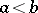) or may be larger () than the latter. The meaning of the operation of addition within each kind of the quantities length, surface area and volume is also generally known. Within each such system of homogeneous quantities the inequalityand the equality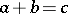have the following properties:

1) for all values ofand all values ofone and only one of the following three relations holds: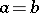oror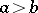;

2) ifand, then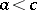(transitivity of the concepts "larger than" and "smaller than" );

3) for any two quantitiesandthere exists a unique quantity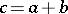;

4)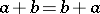(addition is commutative);

5)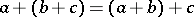(addition is associative);

6)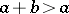(addition is monotone);

7) if, there exists one and only one quantityfor which(possibility of subtraction);

8) for anyand any natural numberthere exists a quantitysuch that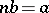(possibility of division);

9) for allandthere exists a natural numbersuch that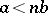. This property is known as the property of Eudoxus or the axiom of Archimedes. It, together with the more elementary properties 1)–8), is implicit to the theory of measuring these quantities, developed by the mathematicians of Ancient Greece.

If some lengthis taken to be the unit, the system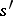of all lengths in a rational relation withsatisfies the requirements 1)–9). The existence of mutually incommensurable segments (the discovery of which is usually credited to the Pythagoreans, 5th century B.C.) shows that the systemdoes not comprise the systemof all possible lengths.

In order to obtain an ultimate theory of quantities, some continuity axiom must be added to the requirements 1)–9), for example:

10) if, for a sequence of quantities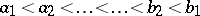, there holds thatfor any quantityand forsufficiently large, then there exists a unique quantitywhich is larger than alland smaller than all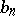.

Properties 1)–10) also define the most modern concept of a system of positive scalar quantities. If some quantityis accepted as the measurement unit in such a system, all other quantities of the system can be uniquely represented as, whereis a positive real number.

II) The study of directed segments on a straight line, velocities with the possibility of two opposite directions and of similar quantities naturally leads to a generalization of the concept of a scalar quantity which is fundamental in mechanics and in physics. The system of scalar quantities thus understood includes not only positive but also negative quantities and a zero. If some positive quantityin such a system is selected as the measurement unit, all other quantities in the system are expressed as, whereis a real number which may be positive, negative or zero. A system of scalar quantities in this sense can obviously also be characterized and defined axiomatically, without basing it on the concept of number. To do this, the requirements 1)–10) defining positive scalar quantities would have to be modified to some extent.

III) In a wider sense of the word, vectors, tensors and other "non-scalars" may also be called "quantities" (cf. Vector; Tensor on a vector space). Such quantities may be added, but the inequality relationbecomes meaningless.

IV) In certain more abstract mathematical studies "non-Archimedean" quantities are considered. They resemble ordinary scalar quantities in that they satisfy the usual inequality requirements, but do not satisfy axiom 9) (this axiom is satisfied for scalar quantities of type II above if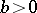).

V) Since the system of positive real numbers satisfies the requirements 1)–10) above, while the system of all real numbers displays all the properties of scalar quantities, it is perfectly legal to give the name of "quantities" to real numbers themselves. This is common practice, in particular, when considering variable quantities. If some given concrete quantity, say the lengthof a heated metal rod, varies with time, then the measured number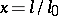varies as well (if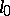remains constant). This time-variable numberis said to be a variable quantity, and it is customary to say thatassumes "numerical values"at some successive moments of time. It is not usual to speak of "variable numbers" in the traditional mathematical terminology, but the following point of view seems logical: numbers, just like lengths, volumes, etc., are particular cases of quantities and, like all quantities, may be both variable and constant. It is just as legitimate to consider variable vectors, tensors, etc., in this way.

Property 1) is also called the trichotomy law. Property 7) is in fact the definition of, and 8) is the definition of homogeneous magnitudes (see Euclid's Elements, Book V, Definition 4).